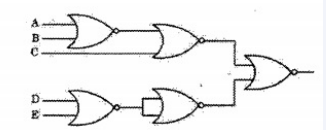# ISRO | ISRO CS 2007 | Question 2

• Last Updated : 20 Nov, 2018

The circuit shown in the following figure realizes the function(A) (( A + B )’ +C ) ( D’E’ )
(B) (( A + B )’ + C ) ( DE’ )
(C) ( A + ( B + C )’ ) ( D’E )
(D) ( A + B + C’ ) ( D’E’ )

Explanation: The given function is equivalent to the following expression:

```Y = (((A + B)' + C)' + ((D + E)')')'
Y = ((A + B)' + C)')' . (D + E)'
Y = ((A + B)' + C) (D'E')```

Option (A) is correct.

Quiz of this Question

My Personal Notes arrow_drop_up